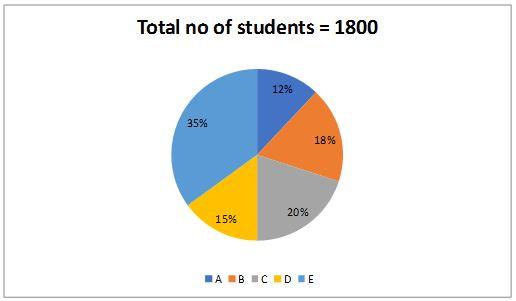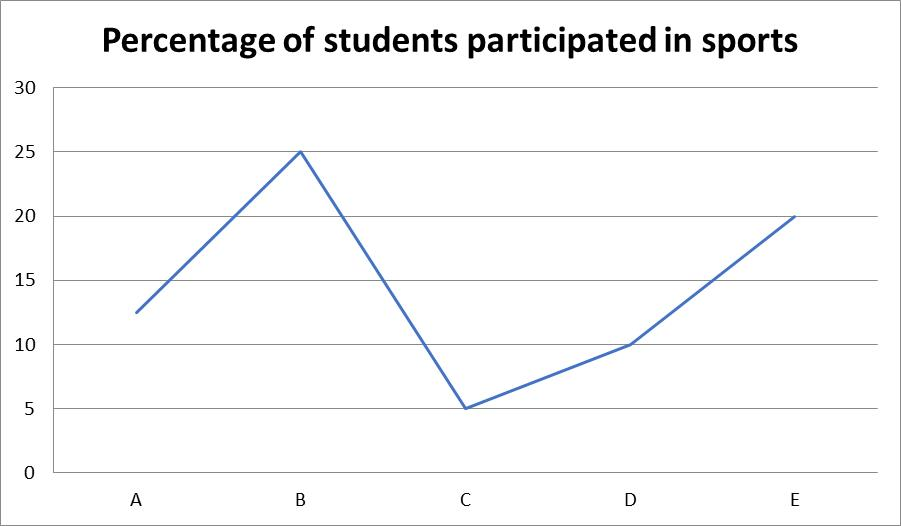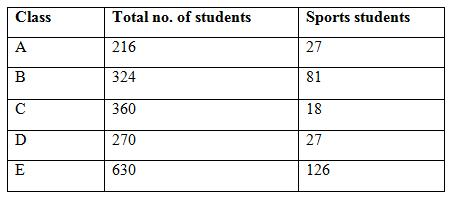# SBI Clerk Mains Quantitative Aptitude (Day-67)

Dear Aspirants, Our IBPS Guide team is providing new series of Quantitative Aptitude Questions for SBI Clerk Mains 2020 so the aspirants can practice it on a daily basis. These questions are framed by our skilled experts after understanding your needs thoroughly. Aspirants can practice these new series questions daily to familiarize with the exact exam pattern and make your preparation effective.

Start Quiz

Ensure Your Ability Before the ExamTake SBI Clerk 2020 Mains Free Mock Test

Directions (01 – 05): Study the following information carefully and answer the questions given below.

Pie chart shows the percentage of students in each class and line graph shows the percentage of students participated in sports.1) What is the ratio of the number of students from E to the students participated in sports from C & D?

A.14: 1

B.1: 14

C.13: 21

D.21: 13

E.None of these

2) What % is the total number of students participated in sports from all the classes together?

A.15.5%

B.17.8%

C.20.3%

D.13.5%

E.None of these

3) Students participating in sports from C & D together is how much % more/less than the no. of students in A?

A.71.72%

B.73.15%

C.79.16%

D.76.18%

E.None of these

4) What is the difference between the no. of students participating sports in A & B together and the total no. of students in D & E together?

A.792

B.996

C.874

D.926

E.None of these

5) In which of the following, the no. of students participating sports is higher?

A.A

B.E

C.B

D.C

E.None of these

Caselet

Directions (06 -10): Study the following information carefully and answer the questions given below.

A, B, C started a business. They invested amounts in the ratio of 1: 2: 3 for 3 months respectively. After this they invested in the ratio of 2: 3: 2 respectively. Investment of A for 6 months is 1600 less than that of C. After 6 months A, B, C invests 2000, 3000 and 4000 respectively for 3 months.

6) If the profit earned by A is 12000, what is the total profit by B and C?

A.46000

B.54000

C.36000

D.42000

E.None of these

7) If B invested 900 for second 3 months, the total profit ratio of A, B & C is

A.34: 55: 70

B.42: 55: 60

C.48: 62: 91

D.42: 34: 91

E.None of these

8) Find the total investment of A if the profit ratio of B to the total profit is 2: 5.

A.12000

B.16000

C.14000

D.18000

E.None of these

9) If the total profit earned by A and C is 32400, what is the amount invested by A, B, C in the second 3 months.

A.3600

B.3200

C.2400

D.2800

E.None of these

10) If A, B and C invests for next 3 months with the double amount they invested in the first 3 months, the profit ratio of A and B becomes 106/179. What is the overall amount invested by A?

A.4600

B.4700

C.4800

D.5700

E.None of these

Directions (01 – 05):Required ratio = 630: 45 => 126: 9 => 14: 1

Required percentage = 279/1800 * 100 = 15.5%

Required percentage = [(18 + 27) – 216]/216 * 100

= 171/216 * 100

= 79.16%

Required difference = 900 – 108 = 792

A – 27

B – 81

C – 18

D – 27

E – 126

Class E has maximum number of students participating in sports.

Directions (06 – 10):

1st 3 months: x: 2x: 3x

2nd 3 months: 2y: 3y: 2y

3rd 3 months: 2000: 3000: 4000

2y + x = (2y + 3x) – 1600

x = 800

So, 1st 3 months: 800: 1600: 2400

To find profit,

(x * 3) + (2y * 3) + 6000: (2x * 3) + (3y * 3) + 9000: (3x * 3) + (2y * 3) + 12000

3x + 6y + 6000: 6x + 9y + 9000: 9x + 6y + 12000

x = 800 so,

6y + 8400: 9y + 13800: 6y + 19200

Now,

A’s profit, 6y + 8400 = 12000

y = 600

B and C’s profit, 9y + 13800 + 6y + 19200 = 5400 + 13800 + 3600 + 19200

= 42000

B: 3y = 900

y = 300

Profit ratio,

(6 * 300 + 8400): (9 * 300 + 13800): (6 * 300 + 19200)

10200: 16500: 21000

Profit ratio of A, B and C = 34: 55: 70

Given ratio = (9y + 13800)/(21y + 41400) = 2/5

45y – 42y = 82800 – 69000

3y = 13800

y = 4600

Now, 800 + (2 * 4600) + 6000 = 16000

A & C’s profit

12y + 27600 = 32400

y = 400

For second 3 months,

2y + 3y + 2y = 7y = 2800

Profit ratio of A and B,

(6y + 8400 + 1600)/(9y + 13800 + 3200) = 106/179

(6y + 10000)/(9y + 17000) = 106/179

y = 100

Overall investment by A,

x + 2y + 2000 + 2x = 800 + 200 + 2000 + 1600 = 4600

 Check Here to View SBI Clerk Mains 2020 Quantitative Aptitude Questions Day 66 Day 65 Day 64 Click Here for SBI Clerk 2020 – Detailed Exam Notification